# Recipient attribute with number value

To create a segment matching a number in an attribute you first select the attribute in the add-pop up.

You'll then define your attribute type as Number

On the segment workspace, click on the dropdown to select the operating criteria: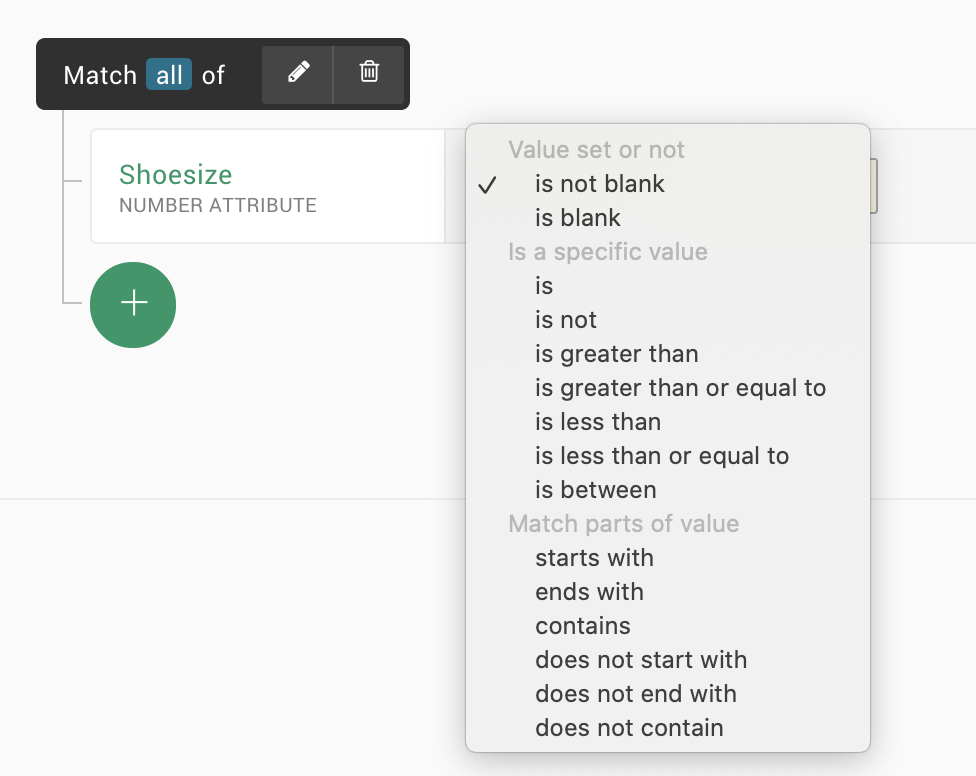• is blank / is not blank
There is no value in the attribute / there is some value in the attribute.
• is less / greater than
• is less /greater than or equal to
The attribute value should be the same or smaller/bigger than your added number.
• is between
The attribute value should be between your added numbers. This will also include the from and to numbers.
• starts with / ends with
The attribute value should be begin/end with your added number(s) (or not).
• contains / does not contain

When you've selected your operating criteria you will need to specify the actual number match (not if you've selected is (not) blank)​; what number(s) value should one contact have in your selected attribute to match your segment?

By adding more than one criteria, any of them should match (not all of them).

The example below shows a segment that matches all contacts with either the value 39 or 8.5 in the attribute Shoesize.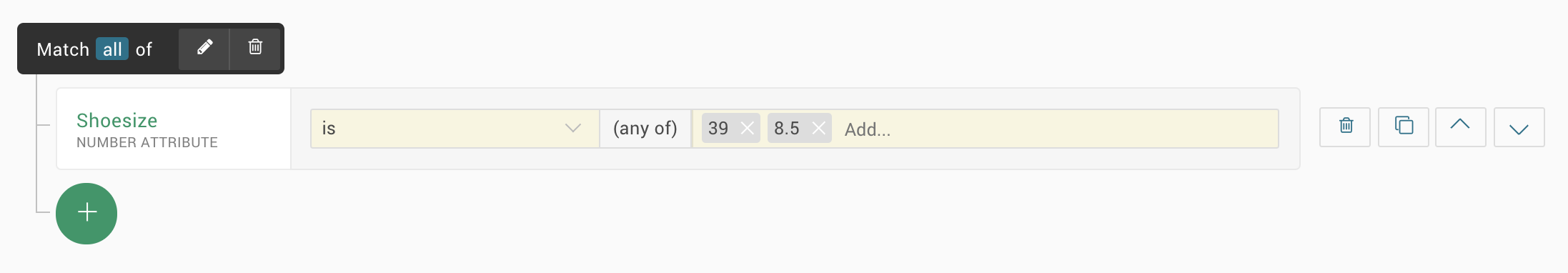Note: this attribute type requires a number value (0-9) and supports period separated decimals (it does not support comma-separated decimals or space).

## Calculating conditions

When adding an attribute with number value you're able to convert the condition to a calculative rule.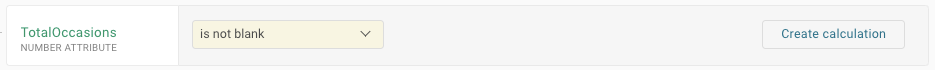By doing so your condition will expand and you'll be able to add another attribute to your calculation.

In the top you'll set your main calculation and the result you want (e.g. A+B=1).

You can calculate using addition, subtraction or division.

• is / is not
The sum of the calculation is exactly the given number / The sum of the calculation is not the given number
• is less than / is greater than
The sum of the calculation is smaller the given number (<X) / The sum of the calculation is bigger the given number (>X)
• is at least / is at most
The sum of the calculation is smaller or equal to the given number (≤X) / The sum of the calculation is bigger or equal the given number (≥X)

Then you specify the values for A and B.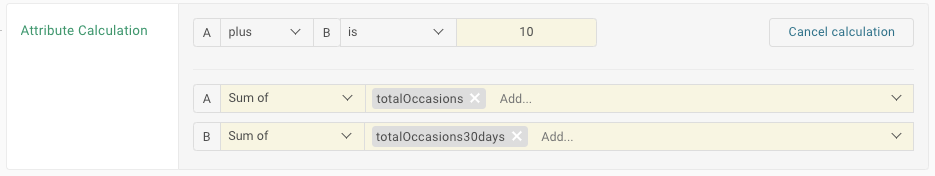You can specify several attributes and select to use the sum of the values in these attributes or the difference between them (the added order your multiple attributes will state how the difference is calculated).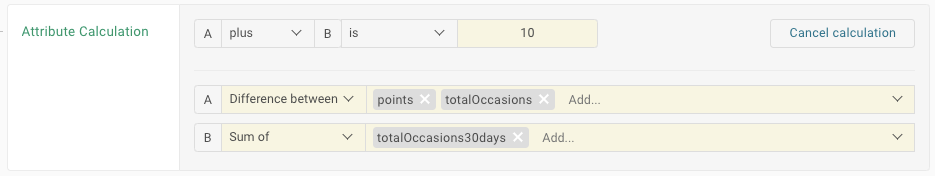A (points-totalOccasions) + B (totalOccasions30days) = 10